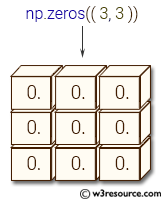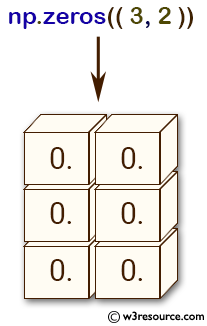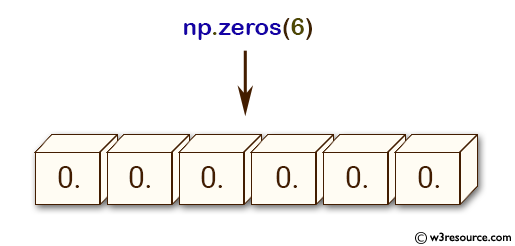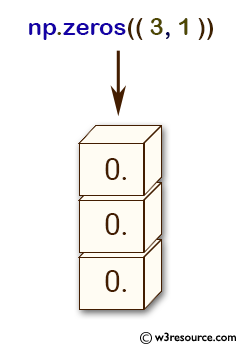# NumPy: numpy.zeros() function

## numpy.zeros() function

The numpy.zeros() function is used to create an array of specified shape and data type, filled with zeros.
The function is commonly used to initialize an array of a specific size and type, before filling it with actual values obtained from some calculations or data sources. It is also used as a placeholder to allocate memory for later use.

Syntax:

`numpy.zeros(a, dtype=None, order='K', subok=True)`Parameters:

Name Description Required /
Optional
shape Shape of the new array, e.g., (2, 3) or 2. Required
dtype The desired data-type for the array, e.g., numpy.int8. Default is numpy.float64. optional
order Whether to store multi-dimensional data in row-major (C-style) or column-major (Fortran-style) order in memory optional

Return value:

[ndarray] Array of zeros with the given shape, dtype, and order.

Example: Creating a numpy array of zeros with a tuple shape

``````>>> import numpy as np
>>> a = (3,2)
>>> np.zeros(a)
array([[ 0.,  0.],
[ 0.,  0.],
[ 0.,  0.]])
``````

In the above code a tuple (3, 2) is created and assigned to variable 'a'. The np.zeros() function is called with 'a' as its argument, which creates a numpy array of zeros with a shape of (3, 2).

Pictorial Presentation:Example: Creating arrays of zeros using NumPy

``````>>> import numpy as np
>>> np.zeros(6)
array([ 0.,  0.,  0.,  0.,  0.,  0.])
>>> np.zeros((6,), dtype=int)
array([0, 0, 0, 0, 0, 0])
>>> np.zeros((3, 1))
array([[ 0.],
[ 0.],
[ 0.]])
``````

In the above code the first line, np.zeros(6) creates a one-dimensional array of size 6 with all elements set to 0, and its data type is float.

In the second line, np.zeros((6,), dtype=int) creates a one-dimensional array of size 6 with all elements set to 0, and its data type is integer.

In the third line, np.zeros((3, 1)) creates a two-dimensional array of size 3x1 with all elements set to 0, and its data type is float.

Pictorial Presentation:Example: Create a 3D array with zeros along a specified axis

Code:

``````import numpy as np

# Create a 3D array with zeros along the second axis
nums = np.zeros((2, 3, 4), dtype=int)
nums[:, 1, :] = 2
print(nums)
``````

Output:

```[[[0 0 0 0]
[2 2 2 2]
[0 0 0 0]]

[[0 0 0 0]
[2 2 2 2]
[0 0 0 0]]]
```

In the above example, we create a 3D array with dimensions (2, 3, 4) using np.zeros() function. We then set the values of the second axis to 2, resulting in a 3D array with zeros along the first and third axis and 2's along the second axis.

Example: Create a 2D array with a custom data type and byte order

Code:

``````import numpy as np

# Create a 2D array of unsigned 16-bit integers with big-endian byte order
nums = np.zeros((2, 3), dtype=np.dtype('>u2'))
print(nums)
``````

Output:

```[[0 0 0]
[0 0 0]]
```

In the above example, we create a 2D array with dimensions (2, 3) using np.zeros() function. Using the np.dtype() function, we specify an unsigned 16-bit integer with big-endian byte order. The resulting array has values of 0 and the specified data type and byte order.

Python - NumPy Code Editor:

Previous: ones_like()
Next: zeros_like()

﻿